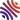## 30 publications1. Positive and sign-changing solutions for a quasilinear Steklov nonlinear boundary problem with critical growth ( and ), In NoDEA Nonlinear Differential Equations Appl., volume 28, .
2. Maximum and antimaximum principles for the $p$-Laplacian with weighted Steklov boundary conditions (, and ), In Electron. J. Differential Equations, .
3. Bifurcation from the first eigenvalue of the $p$-Laplacian with nonlinear boundary condition (, and ), In Electron. J. Differential Equations, .
4. Qualitative results for parabolic equations involving the $p$-Laplacian under dynamical boundary conditions ( and ), In North-West. Eur. J. Math., volume 4, .
5. On abstract indefinite concave-convex problems and applications to quasilinear elliptic equations ( and ), In NoDEA Nonlinear Differential Equations Appl., volume 24, .
6. Superlinear critical resonant problems with small forcing term ( and ), In Calc. Var. Partial Differential Equations, volume 54, . [hal-lmpa]
7. Weighted eigenvalue problems for quasilinear elliptic operators with mixed Robin-Dirichlet boundary conditions ( and ), In J. Math. Anal. Appl., volume 422, .
8. A resonant-superlinear elliptic problem revisited ( and ), In Adv. Nonlinear Stud., volume 13, . [hal-lmpa]
9. Nonlinear eigenvalue problems for degenerate elliptic systems ( and ), In Differential Integral Equations, volume 23, .
10. A one side superlinear Ambrosetti-Prodi problem for the Dirichlet $p$-Laplacian ( and ), In J. Math. Anal. Appl., volume 367, .
11. A weighted eigenvalue problem for the $p$-Laplacian plus a potential ( and ), In NoDEA Nonlinear Differential Equations Appl., volume 16, .
12. An asymmetric Neumann problem with weights (, , and ), In Ann. Inst. H. Poincaré Anal. Non Linéaire, volume 25, .
13. Minimax theorems on $C^1$ manifolds via Ekeland variational principle (), In Abstr. Appl. Anal., .
14. On a resonant-superlinear elliptic problem (, and ), In Calc. Var. Partial Differential Equations, volume 17, .
15. The functional Fučík spectrum has empty interior (, , and ), In Proc. Roy. Soc. Edinburgh Sect. A, volume 133, .
16. Asymmetric elliptic problems with indefinite weights (, , and ), In Ann. Inst. H. Poincaré Anal. Non Linéaire, volume 19, .
17. A nonresonance result for strongly nonlinear second order ODE's ( and ), In Adv. Differential Equations, volume 6, .
18. Eigenvalue problems for the $p$-Laplacian with indefinite weights (), In Electron. J. Differential Equations, .
19. Sur certains problèmes elliptiques asymétriques avec poids indéfinis (, , and ), In C. R. Acad. Sci. Paris Sér. I Math., volume 332, .
20. A nodal domain property for the $p$-Laplacian (, and ), In C. R. Acad. Sci. Paris Sér. I Math., volume 330, .
21. A strong comparison principle for positive solutions of degenerate elliptic equations ( and ), In Differential Integral Equations, volume 13, .
22. The beginning of the Fučik spectrum for the $p$-Laplacian (, and ), In J. Differential Equations, volume 159, .
23. Nonresonance to the right of the first eigenvalue for the one-dimensional $p$-Laplacian (, and ), In Nonlinear Anal., volume 38, .
24. Sur le spectre de Fučik du $p$-Laplacien (, and ), In C. R. Acad. Sci. Paris Sér. I Math., volume 326, .
25. A strong comparison principle for the Dirichlet $p$-Laplacian ( and ), Chapter in Reaction diffusion systems (Trieste, 1995), Dekker, New York, volume 194, .
26. Existence results for quasilinear problems via ordered sub- and supersolutions (), In Ann. Fac. Sci. Toulouse Math. (6), volume 6, .
27. Existence results for resonant perturbations of the Fučik spectrum ( and ), In Topol. Methods Nonlinear Anal., volume 8, .
28. A nonresonance result with respect to the Fučik spectrum for a second order differential equation (), In Differential Integral Equations, volume 9, .
29. A nonresonance result with respect to the Fučík spectrum for a second order differential equation (), Chapter in Partial differential equations (Han-sur-Lesse, 1993), Akademie-Verlag, Berlin, volume 82, .
30. A variational approach to nonresonance with respect to the Fučik spectrum ( and ), In Nonlinear Anal., volume 19, .